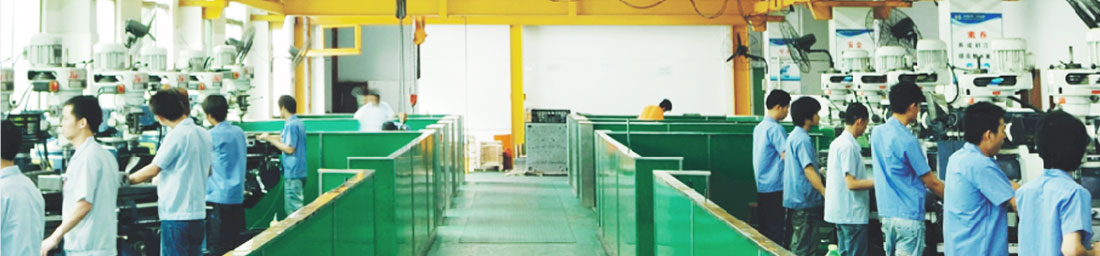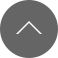# 简单的模具生产流程

Release time:2018-08-25
2D软件开模流程: 画图（2D）==》审图==》放缩水==>镜像==》排版===》拆分零件==》标数==》审图==》出图==》定制模框==》零件加工（最好是单穴，预防结构更改，根据零件的结构需要分别发放到不同的加工部门进行加工）==》经过品质部门检测==》组模==》打样==》品质部门按产品图检测尺寸==》工程部检测产品工程性能(如果不OK,则需要进行改模然后再重新重新组模打样）==》检测OK后进行全模穴模具零件加工==》组模==OK!3D软件开模流程:： 画图（2D）==》审图==》绘制3D产品图==》放缩水==>拆分零件==》转2D零件图==》标数==》审图==》出图==》定制模框==》零件加工（最好是单穴，预防结构更改，根据零件的结构需要分别发放到不同的加工部门进行加工）==》经过品质部门检测==》组模==》打样==》品质部门按产品图检测尺寸==》工程部检测产品工程性能(如果不OK,则需要进行改模然后再重新重新组模打样）==》检测OK后进行全模穴模具零件加工==》组模==OK!